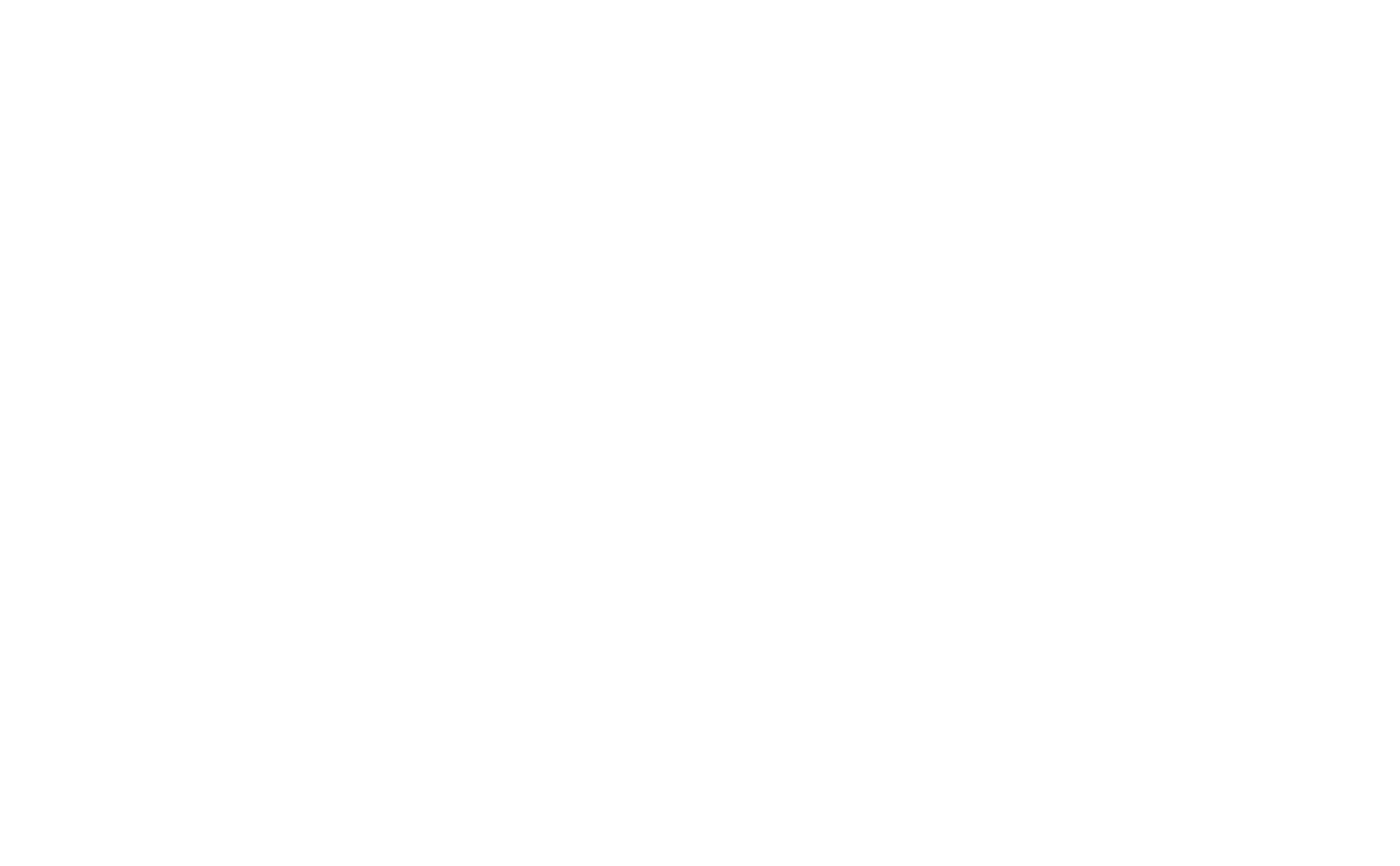Under some conditions, a device and its metadata need to be cleared manually. This can be done with either the new = TRUE argument within the call to Plot. Sometimes, the metadata of a previous plot will prevent correct plotting of a new Plot call. Use clearPlot to clear the device and all the associated metadata manually.

clearPlot(dev = dev.cur(), removeData = TRUE, force = FALSE)

# S4 method for numeric,logical
clearPlot(dev = dev.cur(), removeData = TRUE, force = FALSE)

# S4 method for numeric,missing
clearPlot(dev, force)

# S4 method for missing,logical
clearPlot(removeData, force)

# S4 method for missing,missing
clearPlot(dev = dev.cur(), removeData = TRUE, force = FALSE)

## Arguments

dev

Numeric. Device number to clear.

removeData

Logical indicating whether any data that was stored in the .quickPlotEnv should also be removed; i.e., not just the plot window wiped.

force

Logical or "all". Sometimes the graphics state cannot be fixed by a simple clearPlot(). If TRUE, this will close the device and reopen the same device number. If "all", then all quickPlot related data from all devices will be cleared, in addition to device closing and reopening.

Eliot McIntire

## Examples

library(sp)
library(raster)
library(RColorBrewer)

files <- dir(system.file("maps", package = "quickPlot"), full.names = TRUE, pattern = "tif")
maps <- lapply(files, raster)
names(maps) <- lapply(maps, names)

# put layers into a single stack for convenience
landscape <- stack(maps$DEM, maps$forestCover, maps$forestAge, maps$habitatQuality, maps$percentPine) # can change color palette setColors(landscape, n = 50) <- list(DEM = topo.colors(50), forestCover = brewer.pal(9, "Set1"), forestAge = brewer.pal("Blues", n = 8), habitatQuality = brewer.pal(9, "Spectral"), percentPine = brewer.pal("GnBu", n = 8)) # Make a new raster derived from a previous one; must give it a unique name habitatQuality2 <- landscape$habitatQuality ^ 0.3
names(habitatQuality2) <- "habitatQuality2"

# make a SpatialPoints object
caribou <- sp::SpatialPoints(coords = cbind(x = stats::runif(1e2, -50, 50),
y = stats::runif(1e2, -50, 50)))

# use factor raster to give legends as character strings
ras <- raster(extent(0, 3, 0, 4), vals = sample(1:4, size = 12, replace = TRUE), res = 1)

# needs to have a data.frame with ID as first column - see ?raster::ratify
levels(ras) <- data.frame(ID = 1:4, Name = paste0("Level", 1:4))
Plot(ras, new = TRUE)

# Arbitrary values for factors, including zero and not all levels represented in raster
levs <- c(0:5, 7:12)
ras <- raster(extent(0, 3, 0, 2), vals = c(1, 1, 3, 5, 8, 9), res = 1)
levels(ras) <- data.frame(ID = levs, Name = LETTERS[c(1:3, 8:16)])
Plot(ras, new = TRUE)

# Arbitrary values for factors, including zero and not all levels represented in raster
levs <- c(0:5, 7:23)
ras <- raster(extent(0, 3, 0, 2), vals = c(1, 1, 3, 5, 8, 9), res = 1)
levels(ras) <- data.frame(ID = levs, Name = LETTERS[1:23])
Plot(ras, new = TRUE)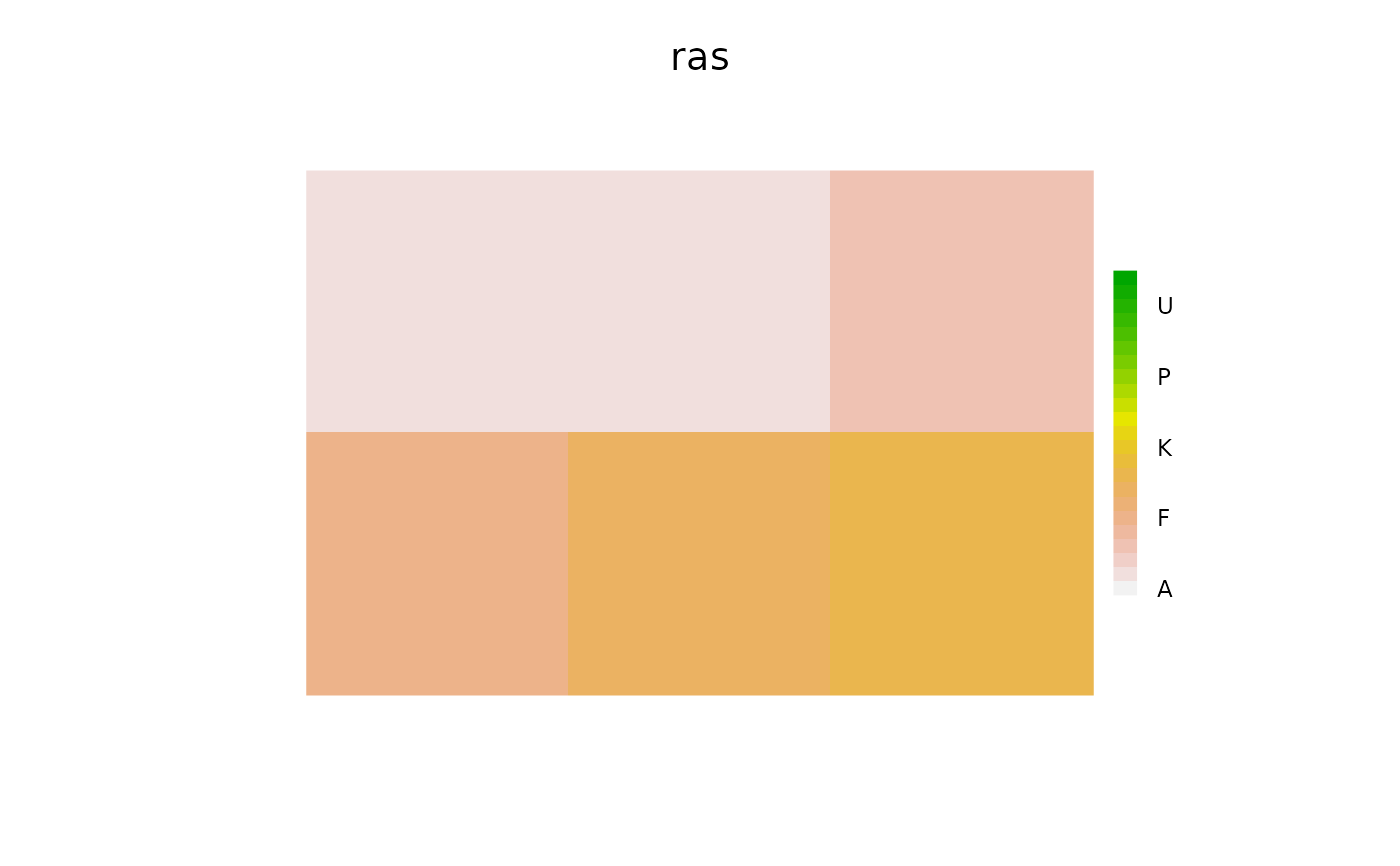# SpatialPolygons
sr1 <- sp::Polygon(cbind(c(2, 4, 4, 1, 2), c(2, 3, 5, 4, 2)) * 20 - 50)
sr2 <- sp::Polygon(cbind(c(5, 4, 2, 5), c(2, 3, 2, 2)) * 20 - 50)
srs1 <- sp::Polygons(list(sr1), "s1")
srs2 <- sp::Polygons(list(sr2), "s2")
spP <- sp::SpatialPolygons(list(srs1, srs2), 1:2)

clearPlot()
Plot(ras)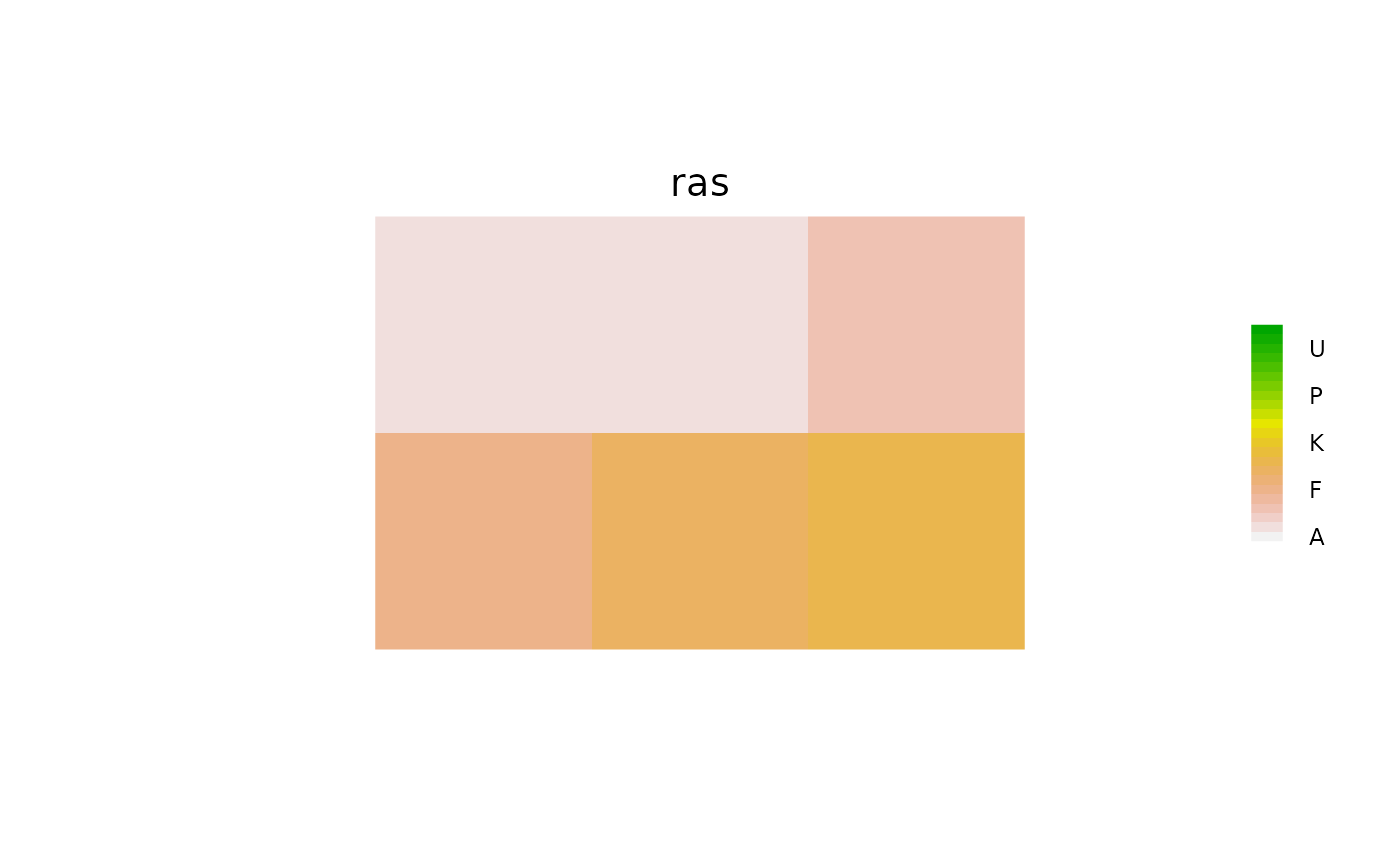clearPlot()
Plot(landscape)

Plot(caribou, addTo = "landscape$forestAge", size = 4, axes = FALSE) # can add a plot to the plotting window Plot(caribou, new = FALSE)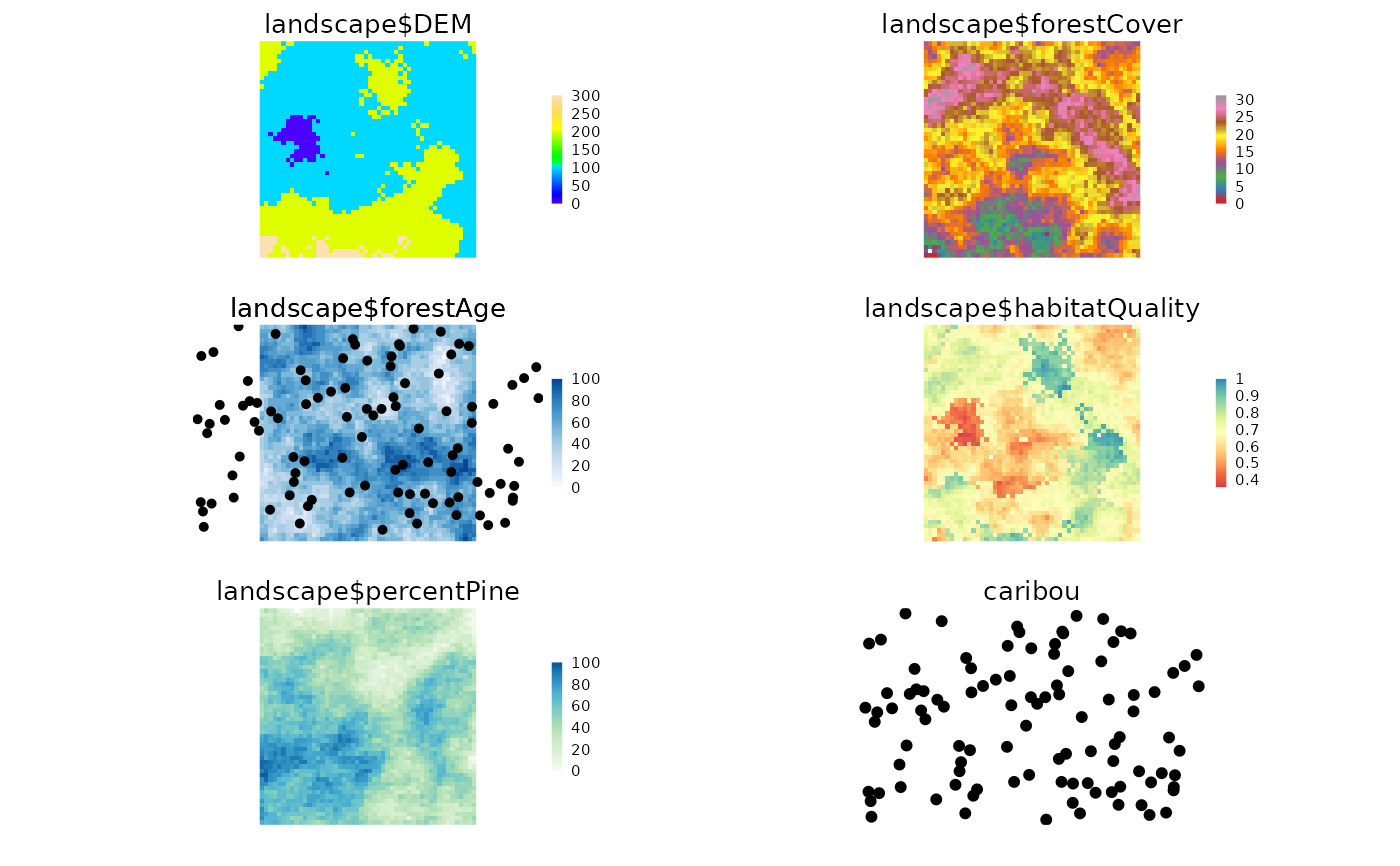# Can add two maps with same name, if one is in a stack; they are given # unique names based on object name Plot(landscape, caribou, maps$DEM)

# can mix stacks, rasters, SpatialPoint*
Plot(landscape, habitatQuality2, caribou)

# can mix stacks, rasters, SpatialPoint*, and SpatialPolygons*
Plot(landscape, caribou)
Plot(habitatQuality2, new = FALSE)
Plot(spP)
Plot(spP, addTo = "landscape$forestCover", gp = gpar(lwd = 2)) # provide arrangement, NumRow, NumCol Plot(spP, arr = c(1, 4), new = TRUE)# example base plot clearPlot() Plot(1:10, 1:10, addTo = "test", new = TRUE) # if there is no "test" then it will make it Plot(4, 5, pch = 22, col = "blue", addTo = "test")obj1 <- rnorm(1e2) Plot(obj1, axes = "L")# Can plot named lists of objects (but not base objects yet) ras1 <- ras2 <- ras a <- list() for (i in 1:2) { a[[paste0("ras", i)]] <- get(paste0("ras", i)) } a$spP <- spP
clearPlot()
Plot(a)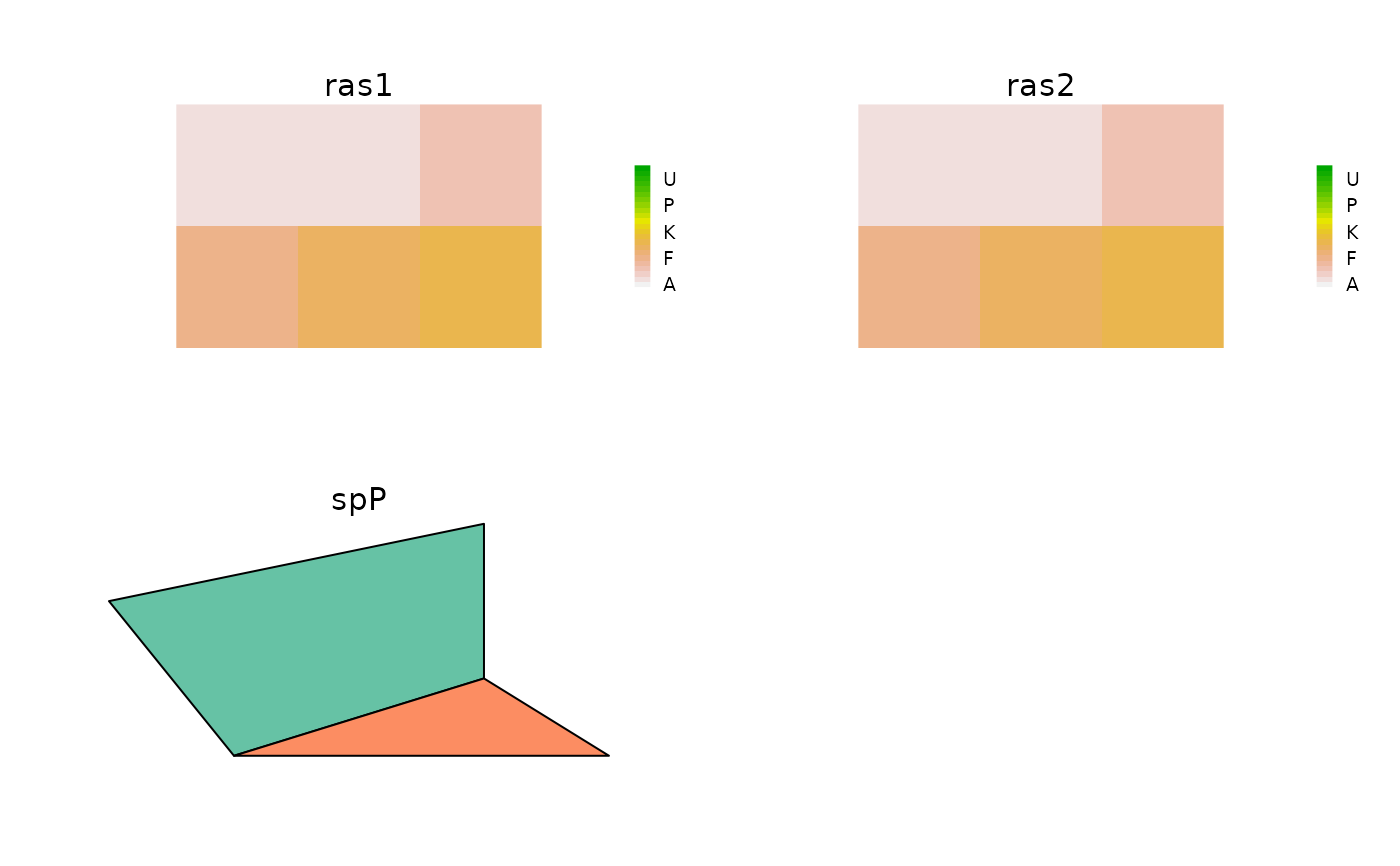# clean up
clearPlot()